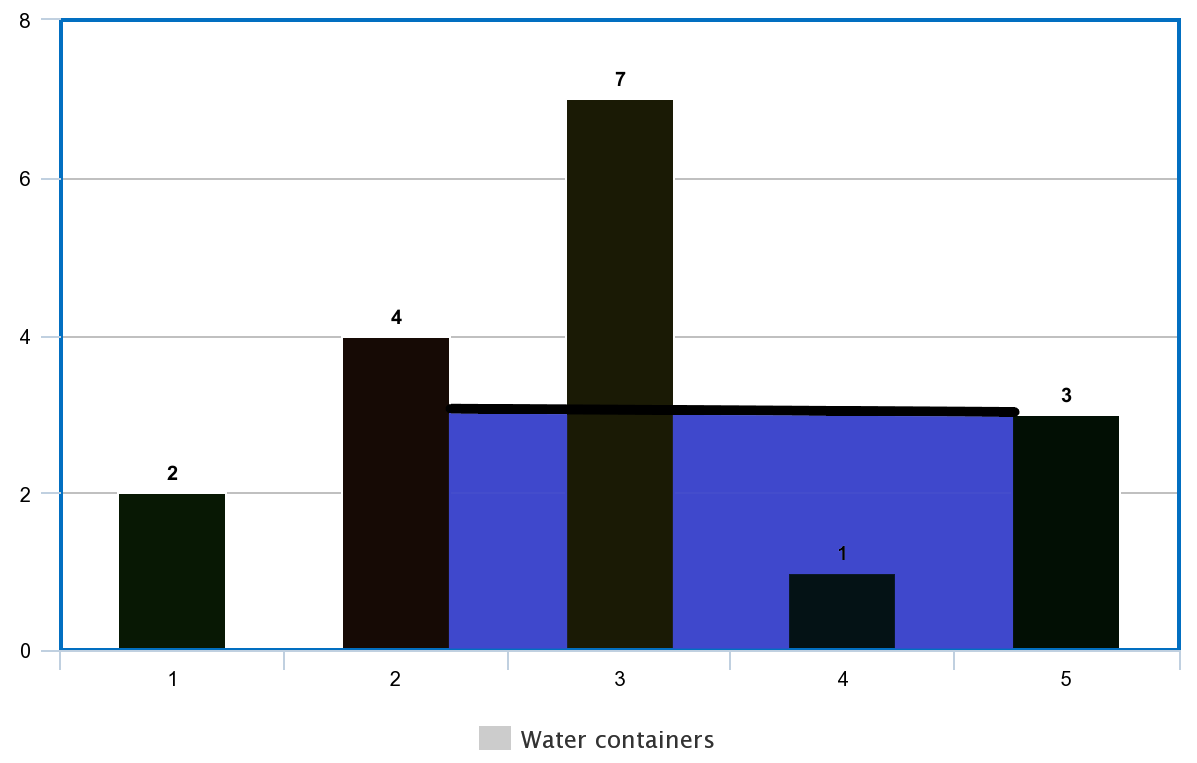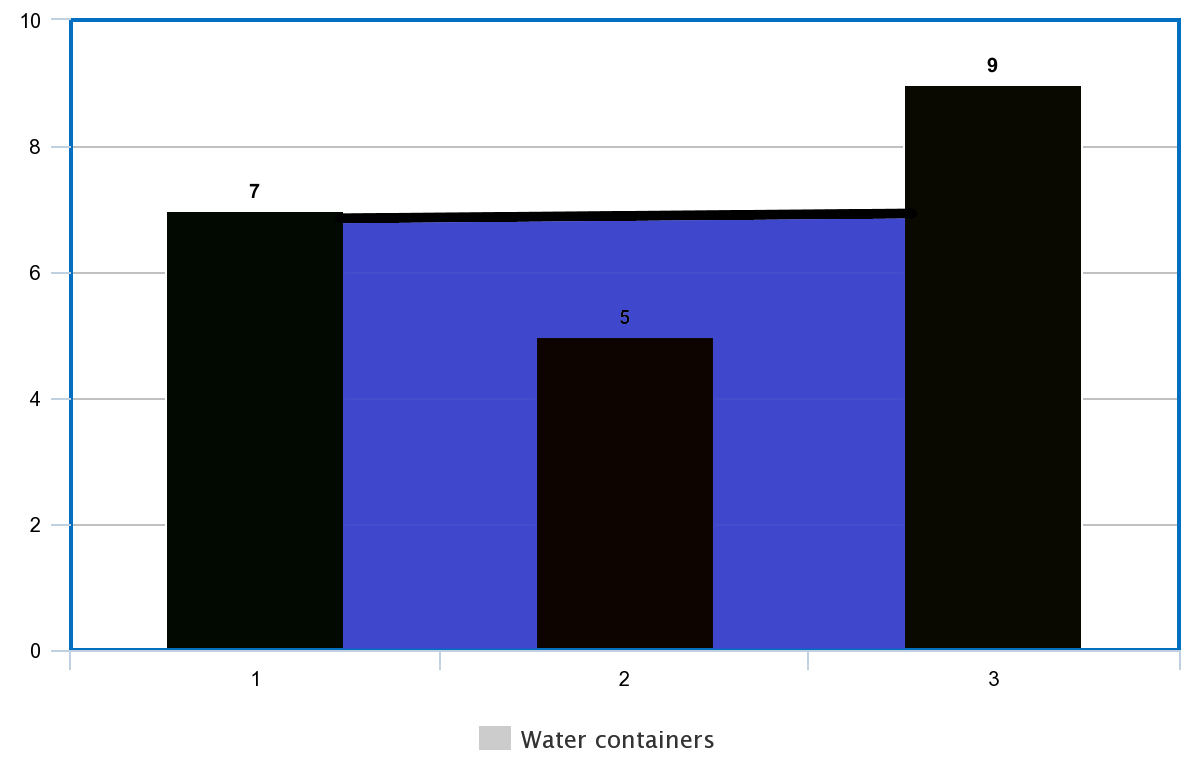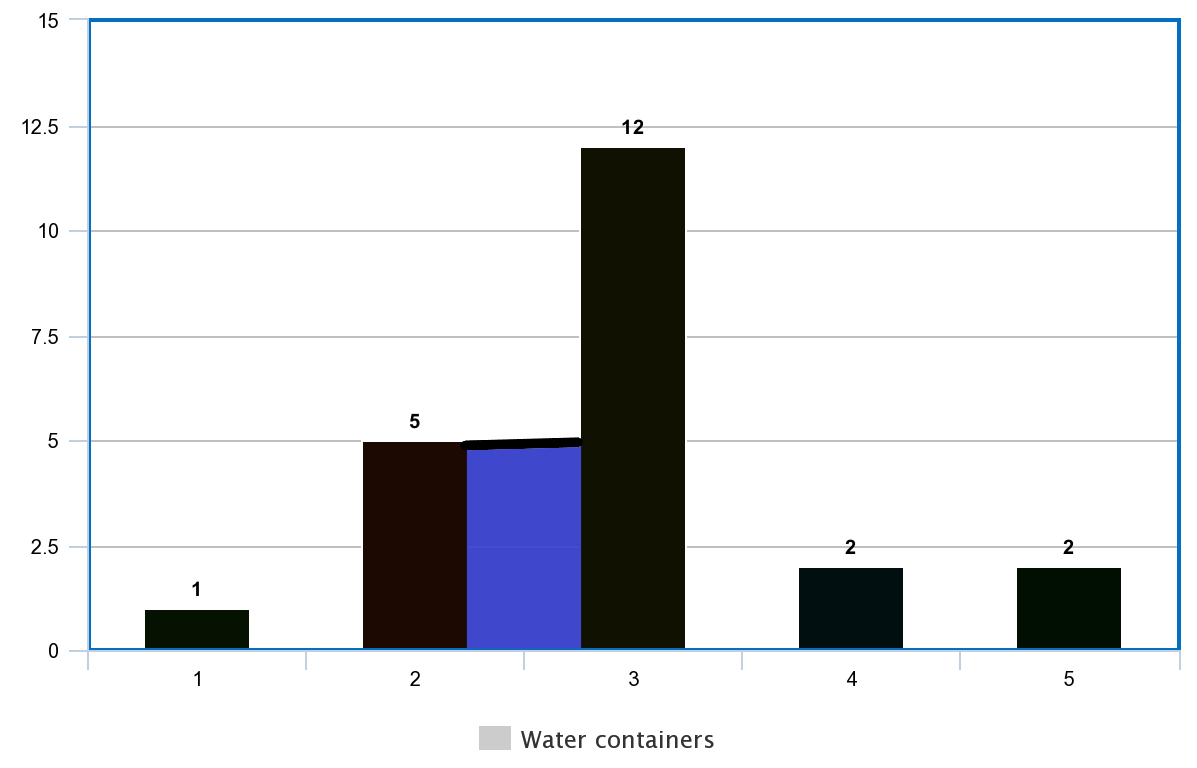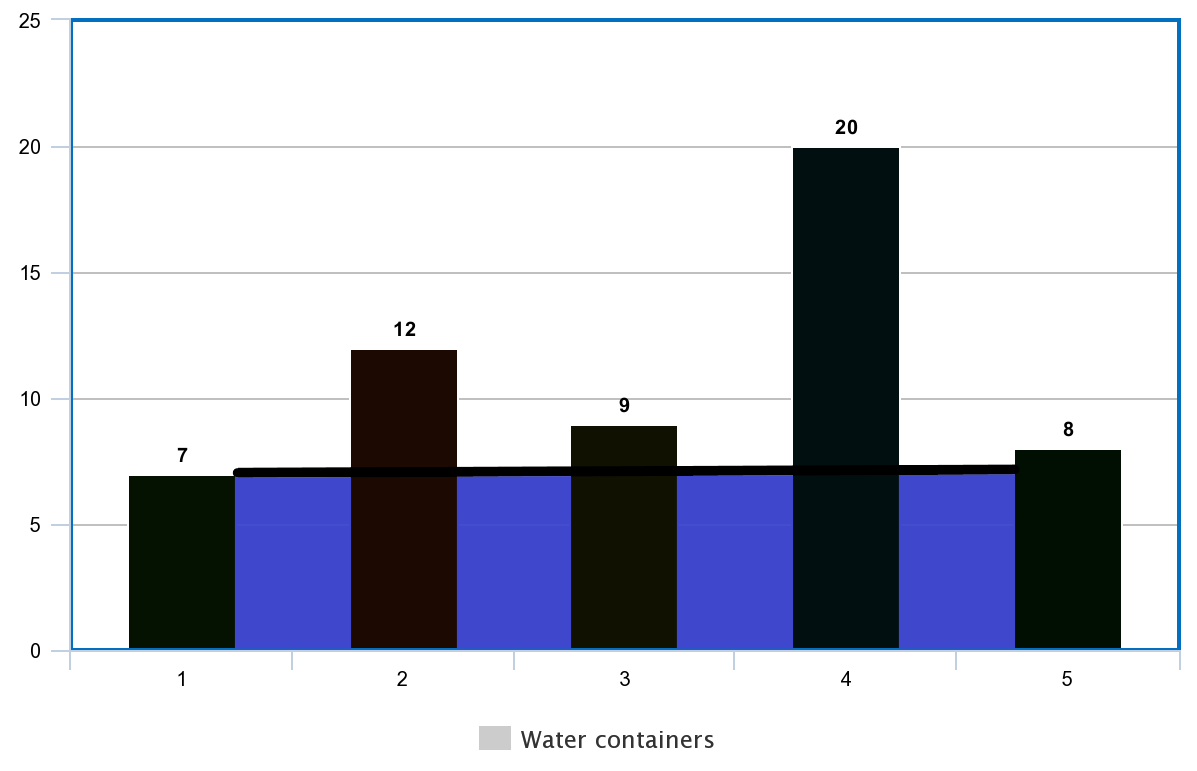6

# Container with Maximum Water

Difficulty: EASYContributed By
Deep Mavani
Avg. time to solve
10 min
Success Rate
90%

Problem Statement

#### Your task is to find two lines, which, together with the x-axis, form a container, such that the container contains the most water. Return the maximum area of the container.

##### Note:
``````1. Consider the container to be 2-dimensional for simplicity.
2. For any pair of sides ignore all the lines that lie inside that pair of sides.
3. You are not allowed to slant the container.
``````#### Example:

``````Consider 'ARR'= {1,8,6,2,5,4,8,3,7} then the vertical lines are represented by the above image. The blue section denotes the maximum water than a container can contain.
``````
##### Input Format:
``````The first line contains a single integer ‘T’ denoting the number of test cases.

The first line of each test case contains a single integer ‘N’ denoting the number of elements in the array/list.

The second line of each test case contains ‘N’ single space-separated integers denoting the elements of the array/list.
``````
##### Output Format:
``````For each test case, return a single integer which denotes the maximum area of the container.
``````
##### Note:
``````You don’t need to print the output, It has already been taken care of. Just implement the given function.
``````
##### Constraints:
``````1 <= T <= 100
2 <= N <= 5000
1 <= ARR[i] <= 10^5

Where 'ARR[i]' denotes the elements of the given array/list.

Time limit: 1 sec
``````
##### Sample Input 1 :
``````2
5
2 4 7 1 3
3
7 5 9
``````
##### Sample Output 1:
``````9
14
``````
##### Explanation of Sample Input 1:``````In the first test case, we will get the maximum area if we choose the container coloured blue in the above image. The length of the base of the container is 3, and the height of the container is min(4, 3) which is 3. Thus, the area of the container is 3 * 3 = 9.
````````````In the second test case, we will get the maximum area if we choose the container coloured blue in the above image. The length of the base of the container is 2, and the height of the container is min(7, 9) which is 7. Thus, the area of the container is 2 * 7 = 14.
``````
##### Sample Input 2:
``````2
5
1 5 12 2 1
5
7 12 9 20 8
``````
##### Sample Output 2:
``````5
28
``````
##### Explanation of Sample Input 2:`````` In the first test case, we will get the maximum area if we choose the container coloured blue in the above image. The length of the base of the container is 1, and the height of the container is min(5, 12) which is 5. Thus, the area of the container is 1 * 5 = 5.
````````````In the second test case, we will get the maximum area if we choose the container coloured blue in the above image. The length of the base of the container is 4, and the height of the container is min(7, 8) which is 7. Thus, the area of the container is 4 * 7 = 28.
``````Console